# Electric Field The Concept of the Electric Field

• Slides: 47Electric FieldThe Concept of the Electric Field In the force model of the electric field, the positive charge A exerts an attractive force on charge B. © 2015 Pearson Education, Inc.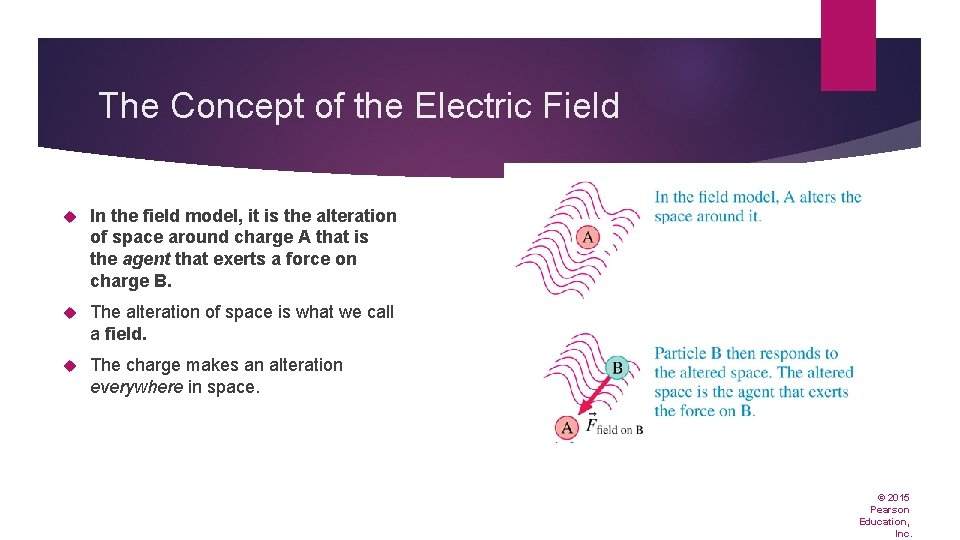The Concept of the Electric Field In the field model, it is the alteration of space around charge A that is the agent that exerts a force on charge B. The alteration of space is what we call a field. The charge makes an alteration everywhere in space. © 2015 Pearson Education, Inc.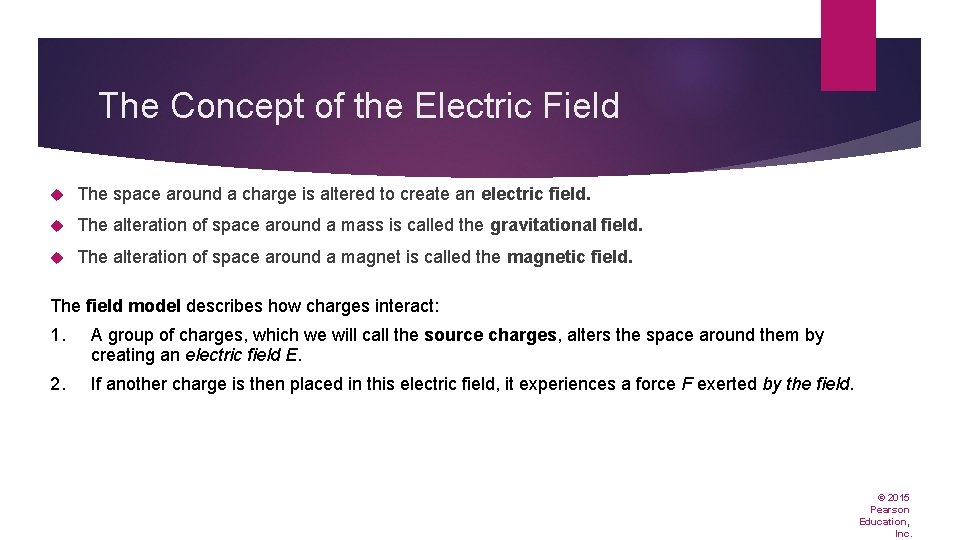The Concept of the Electric Field The space around a charge is altered to create an electric field. The alteration of space around a mass is called the gravitational field. The alteration of space around a magnet is called the magnetic field. The field model describes how charges interact: 1. A group of charges, which we will call the source charges, alters the space around them by creating an electric field E. 2. If another charge is then placed in this electric field, it experiences a force F exerted by the field. © 2015 Pearson Education, Inc.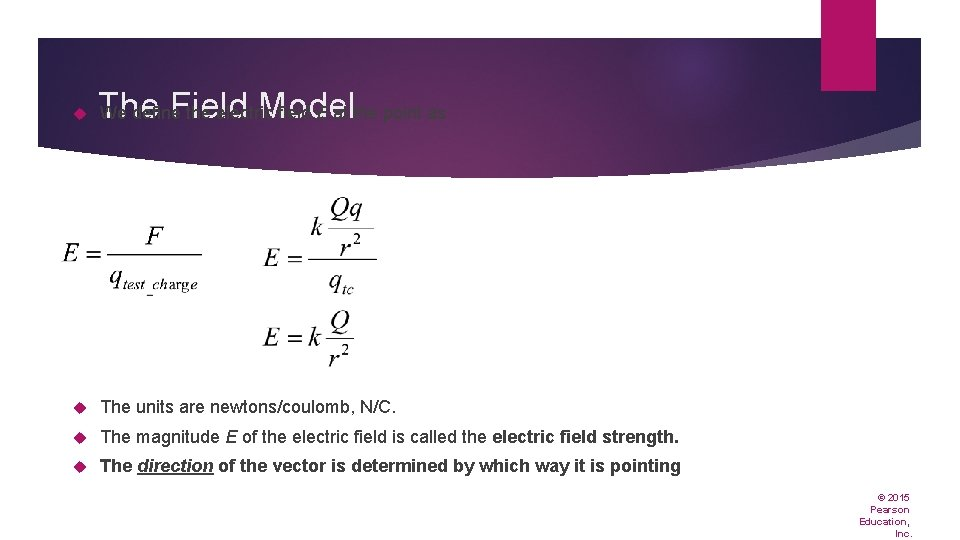The Field Model We define the electric field E at the point as The units are newtons/coulomb, N/C. The magnitude E of the electric field is called the electric field strength. The direction of the vector is determined by which way it is pointing © 2015 Pearson Education, Inc.The Field Model In the field model, the field is the agent that exerts an electric force on a particle with charge q. 1. 2. 3. 4. The electric field, a vector, exists at every point in space. Electric field diagrams will show a sample of vectors, but there is an electric field vector at every point whether one is shown or not. the electric field vector points AWAY from a POSITVE charge source the electric field vector points TOWARD a NEGATIVE charge source The electric field does not depend on the magnitude of the charge used to probe the field (test charge). The electric field depends only on the source charges that create the field. © 2015 Pearson Education, Inc.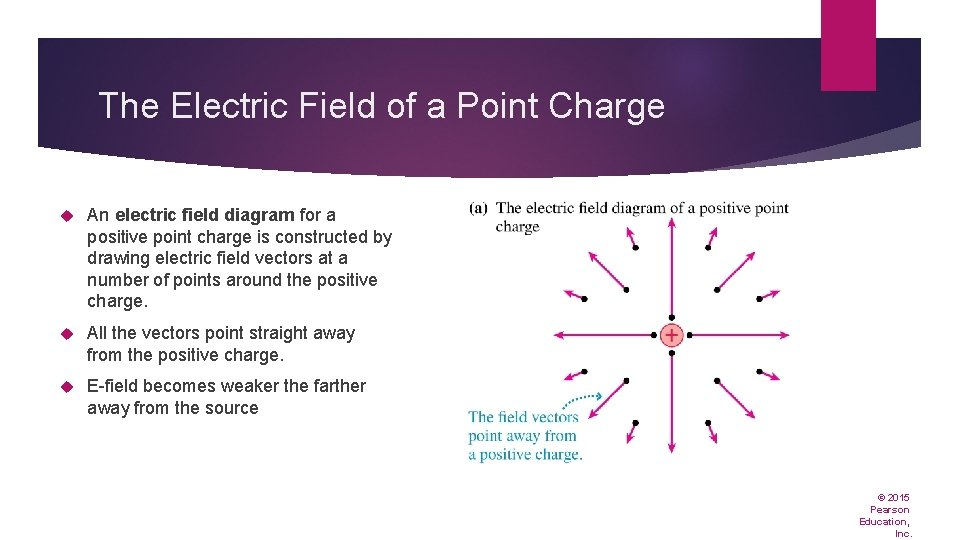The Electric Field of a Point Charge An electric field diagram for a positive point charge is constructed by drawing electric field vectors at a number of points around the positive charge. All the vectors point straight away from the positive charge. E-field becomes weaker the farther away from the source © 2015 Pearson Education, Inc.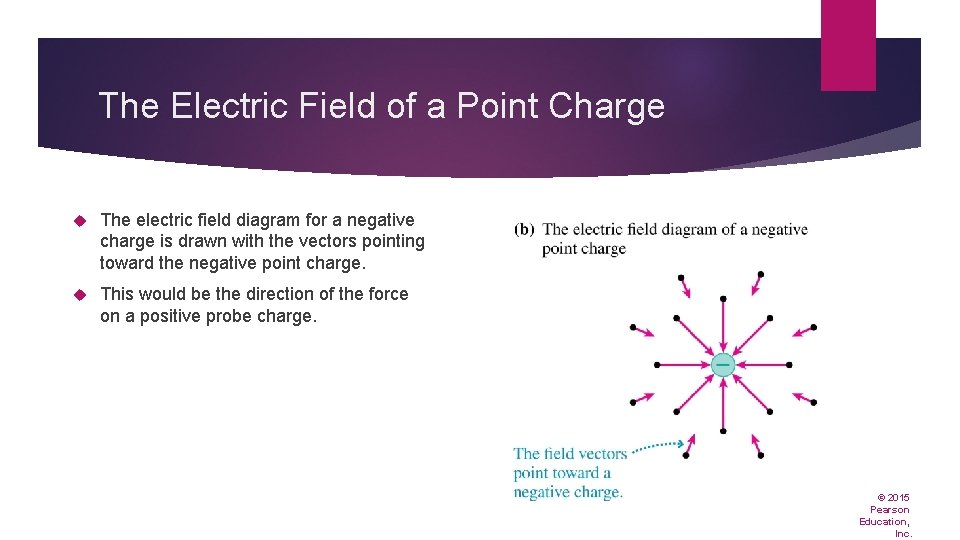The Electric Field of a Point Charge The electric field diagram for a negative charge is drawn with the vectors pointing toward the negative point charge. This would be the direction of the force on a positive probe charge. © 2015 Pearson Education, Inc.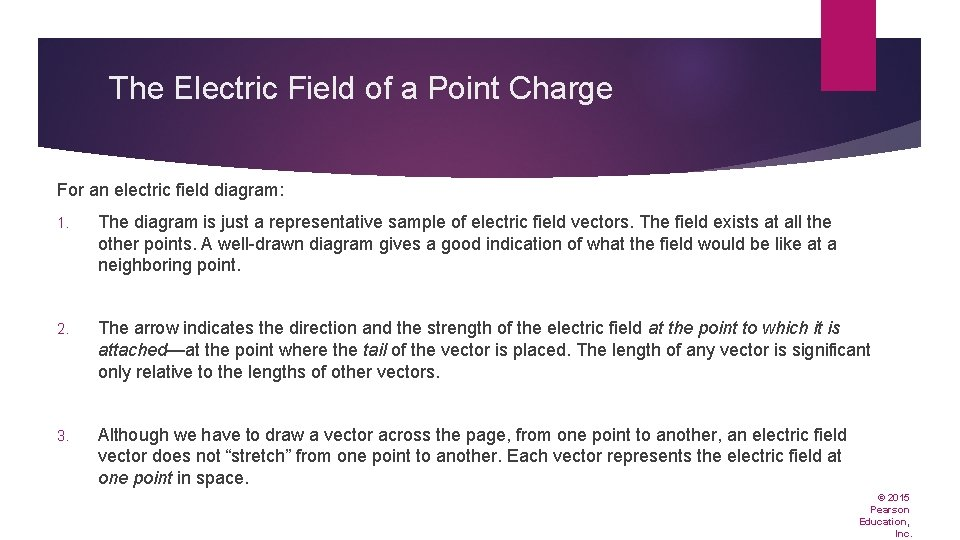The Electric Field of a Point Charge For an electric field diagram: 1. The diagram is just a representative sample of electric field vectors. The field exists at all the other points. A well-drawn diagram gives a good indication of what the field would be like at a neighboring point. 2. The arrow indicates the direction and the strength of the electric field at the point to which it is attached—at the point where the tail of the vector is placed. The length of any vector is significant only relative to the lengths of other vectors. 3. Although we have to draw a vector across the page, from one point to another, an electric field vector does not “stretch” from one point to another. Each vector represents the electric field at one point in space. © 2015 Pearson Education, Inc.Example Problem A small bead, sitting at the origin, has a charge of +10 n. C. At the point (3. 0 cm, 4. 0 cm), what is the magnitude and direction of the electric field due to this bead? © 2015 Pearson Education, Inc.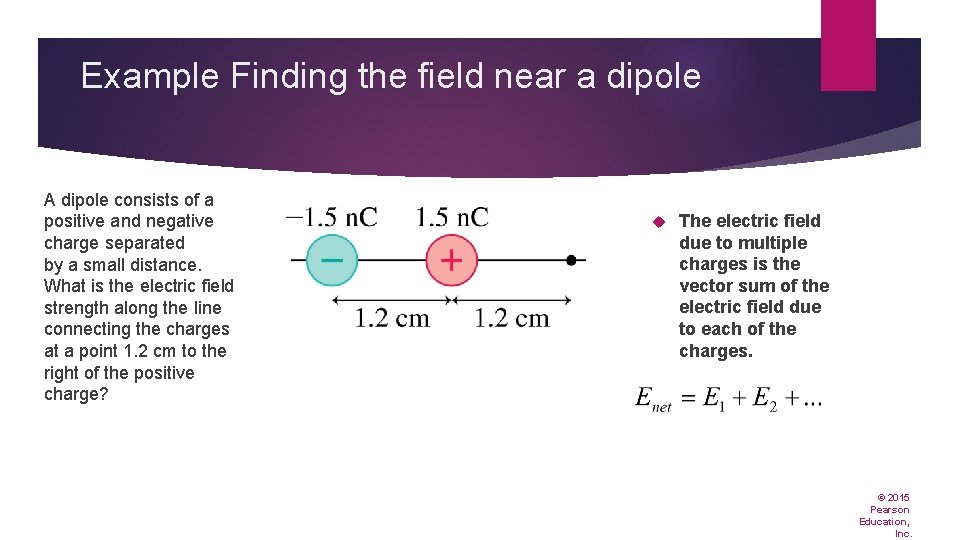Example Finding the field near a dipole A dipole consists of a positive and negative charge separated by a small distance. What is the electric field strength along the line connecting the charges at a point 1. 2 cm to the right of the positive charge? The electric field due to multiple charges is the vector sum of the electric field due to each of the charges. © 2015 Pearson Education, Inc.Example Problem Determine the magnitude and the direction of the electric field at point A. © 2015 Pearson Education, Inc.Uniform Electric Fields A parallel-plate capacitor is the arrangement of two electrodes closely spaced and charged equally but oppositely. An electrode is a conducting plate. © 2015 Pearson Education, Inc.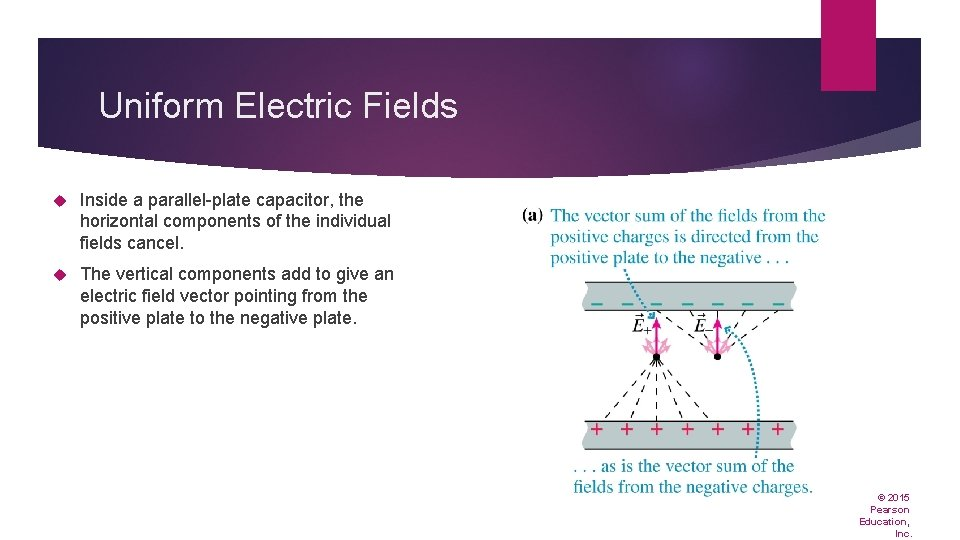Uniform Electric Fields Inside a parallel-plate capacitor, the horizontal components of the individual fields cancel. The vertical components add to give an electric field vector pointing from the positive plate to the negative plate. © 2015 Pearson Education, Inc.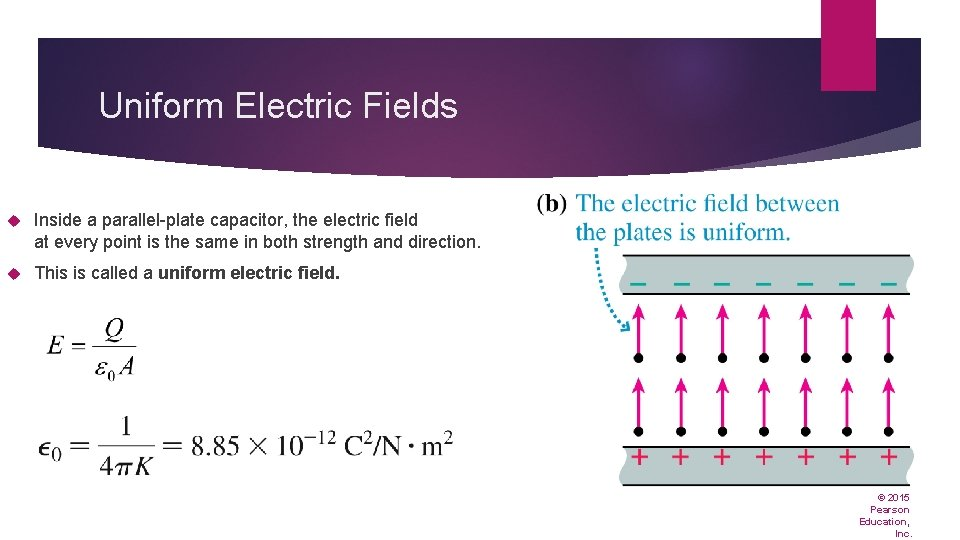Uniform Electric Fields Inside a parallel-plate capacitor, the electric field at every point is the same in both strength and direction. This is called a uniform electric field. © 2015 Pearson Education, Inc.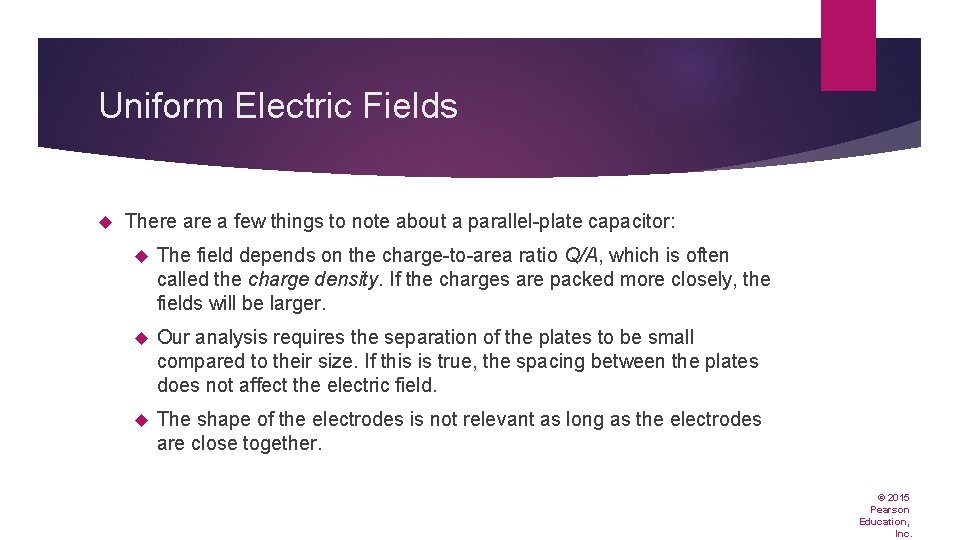Uniform Electric Fields There a few things to note about a parallel-plate capacitor: The field depends on the charge-to-area ratio Q/A, which is often called the charge density. If the charges are packed more closely, the fields will be larger. Our analysis requires the separation of the plates to be small compared to their size. If this is true, the spacing between the plates does not affect the electric field. The shape of the electrodes is not relevant as long as the electrodes are close together. © 2015 Pearson Education, Inc.Example: Finding the field in an air cleaner Long highway tunnels must have air cleaners to remove dust and soot coming from passing cars and trucks. In one type, known as an electrostatic precipitator, air passes between two oppositely charged metal plates, as in FIGURE 20. 31. © 2015 Pearson Education, Inc.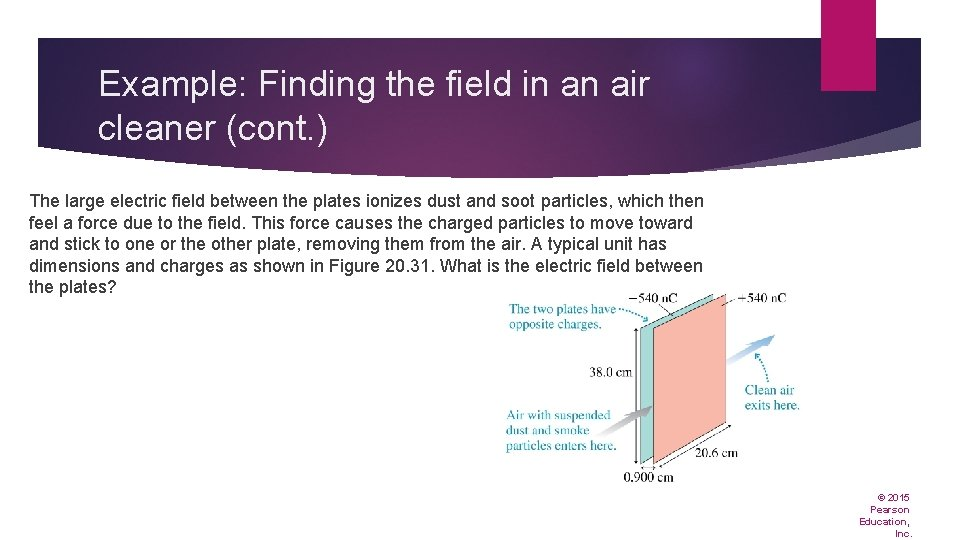Example: Finding the field in an air cleaner (cont. ) The large electric field between the plates ionizes dust and soot particles, which then feel a force due to the field. This force causes the charged particles to move toward and stick to one or the other plate, removing them from the air. A typical unit has dimensions and charges as shown in Figure 20. 31. What is the electric field between the plates? © 2015 Pearson Education, Inc.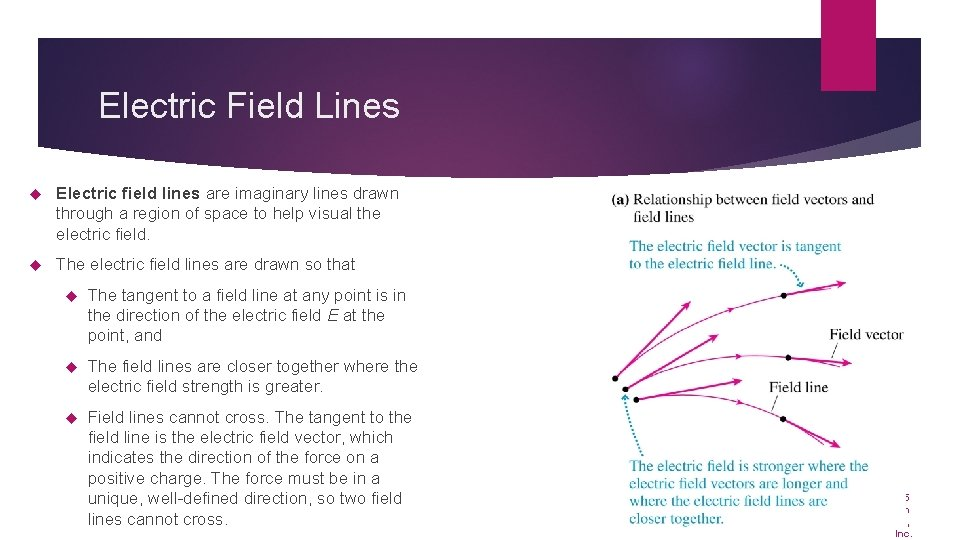Electric Field Lines Electric field lines are imaginary lines drawn through a region of space to help visual the electric field. The electric field lines are drawn so that The tangent to a field line at any point is in the direction of the electric field E at the point, and The field lines are closer together where the electric field strength is greater. Field lines cannot cross. The tangent to the field line is the electric field vector, which indicates the direction of the force on a positive charge. The force must be in a unique, well-defined direction, so two field lines cannot cross. © 2015 Pearson Education, Inc.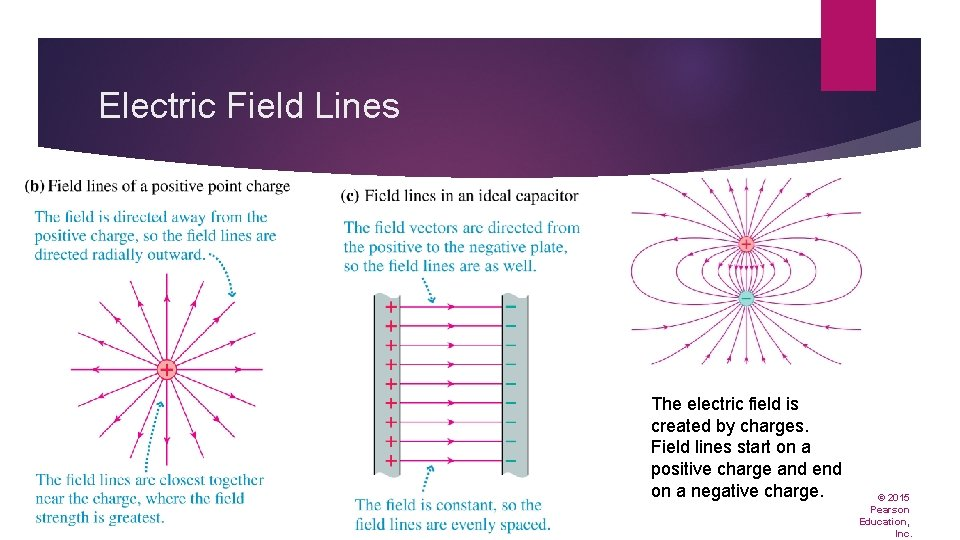Electric Field Lines The electric field is created by charges. Field lines start on a positive charge and end on a negative charge. © 2015 Pearson Education, Inc.Conceptual Example 20. 9 Drawing electric field lines for a charged sphere and a plate FIGURE 20. 36 shows a positively charged metal sphere above a conducting plate with a negative charge. Sketch the electric field lines. © 2015 Pearson Education, Inc.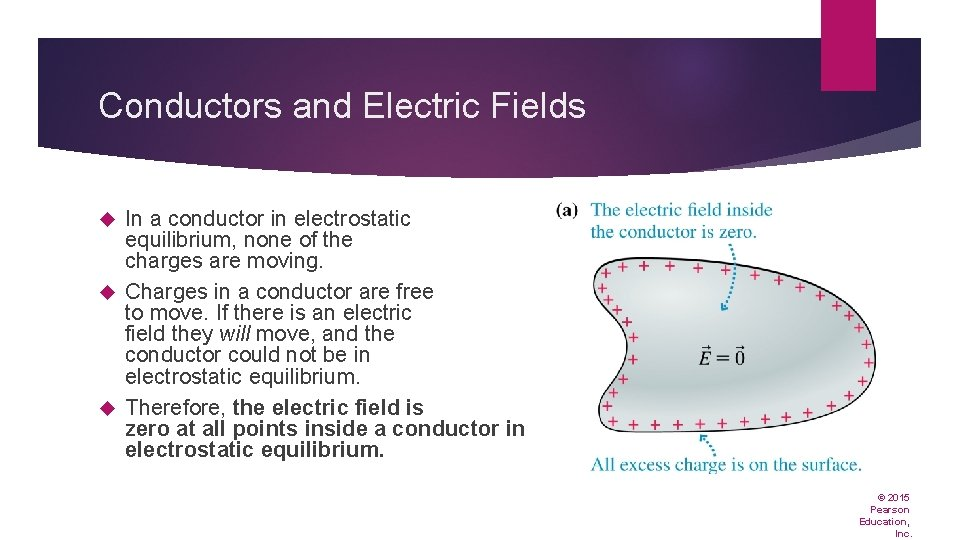Conductors and Electric Fields In a conductor in electrostatic equilibrium, none of the charges are moving. Charges in a conductor are free to move. If there is an electric field they will move, and the conductor could not be in electrostatic equilibrium. Therefore, the electric field is zero at all points inside a conductor in electrostatic equilibrium. © 2015 Pearson Education, Inc.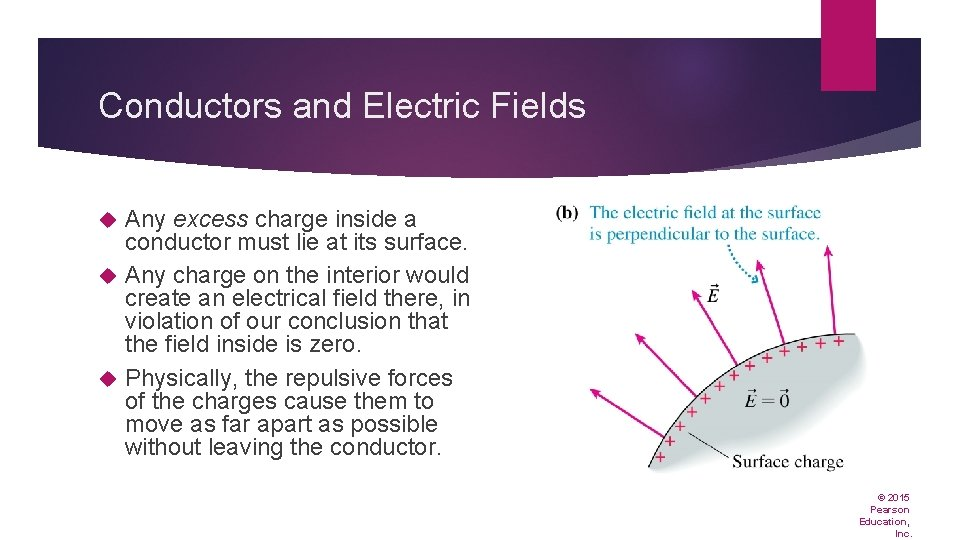Conductors and Electric Fields Any excess charge inside a conductor must lie at its surface. Any charge on the interior would create an electrical field there, in violation of our conclusion that the field inside is zero. Physically, the repulsive forces of the charges cause them to move as far apart as possible without leaving the conductor. © 2015 Pearson Education, Inc.Conductors and Electric Fields The electric field right at the surface of a charged conductor is perpendicular to the surface. If the electric field had a component tangent to the surface, it would exert a force on charges at the surface and cause them to move along the surface, violating the assumption that all charges are at rest. © 2015 Pearson Education, Inc.Conductors and Electric Fields The electric field within a conducting enclosure is zero. A conducting box can be used to exclude electric fields from a region of space; this is called screening. Metal walls are ideal for screening, but wire screens or wire mesh can be used. © 2015 Pearson Education, Inc.Conductors and Electric Fields Although any excess charge of a conductor will be found on the surface, it may not be uniformly distributed. At sharp points, the density of the charge is higher, and therefore the electric field is stronger. © 2015 Pearson Education, Inc.Conductors and Electric Fields The electric field near very sharp points may be strong enough to ionize the air around it. A lightning rod has a sharp point so that if a building is beginning to accumulate charge, meaning a lightning strike might be imminent, a large field develops at the tip of the rod. Once the field ionizes the air, excess charge from the building can dissipate into the air, reducing the electric field and the likelihood of a lightning strike. The lightning rod is intended to prevent a lightning strike. © 2015 Pearson Education, Inc.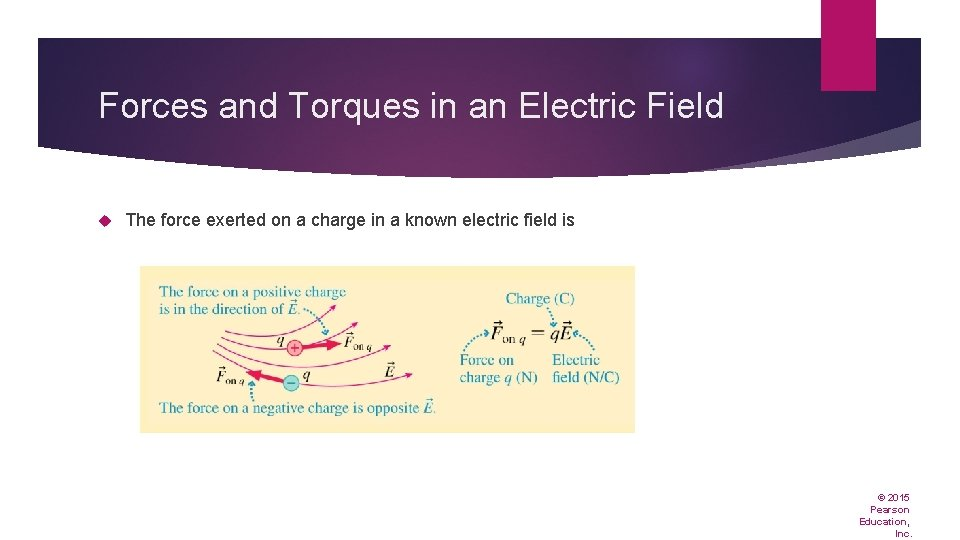Forces and Torques in an Electric Field The force exerted on a charge in a known electric field is © 2015 Pearson Education, Inc.Example: Finding the force on an electron in the atmosphere Under normal circumstances, the earth’s electric field outdoors near ground level is uniform, about 100 N/C, directed down. What is the electric force on a free electron in the atmosphere? What acceleration does this force cause? © 2015 Pearson Education, Inc.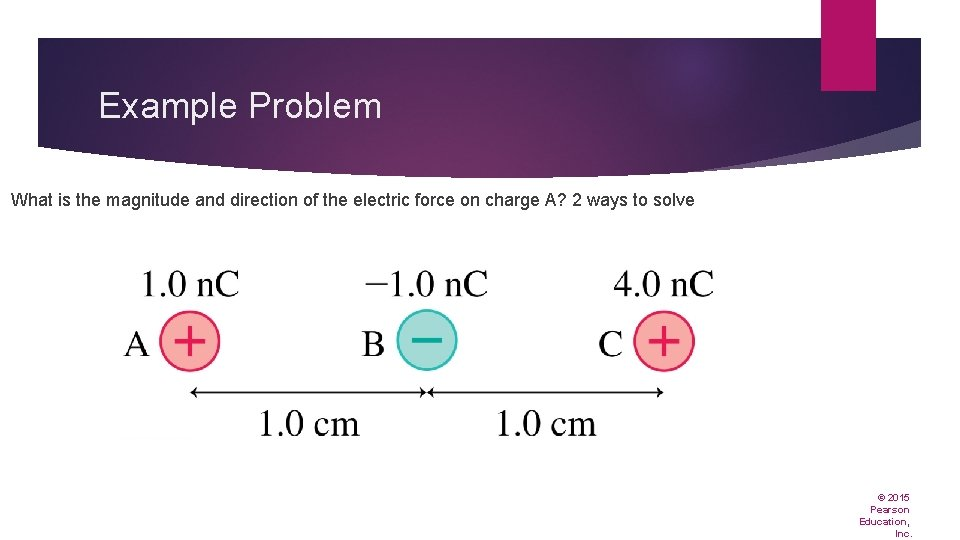Example Problem What is the magnitude and direction of the electric force on charge A? 2 ways to solve © 2015 Pearson Education, Inc.Forces and Torques in an Electric Field An electric dipole in a uniform electric field experiences no net force. There is a net torque on a dipole in a uniform electric field, which causes it to rotate. The electric dipole moment is a vector pointing from the negative to the positive charge of a dipole. © 2015 Pearson Education, Inc.Forces and Torques in an Electric Field The equilibrium position of a dipole in an electric field is with the electric dipole moment aligned with the field. © 2015 Pearson Education, Inc.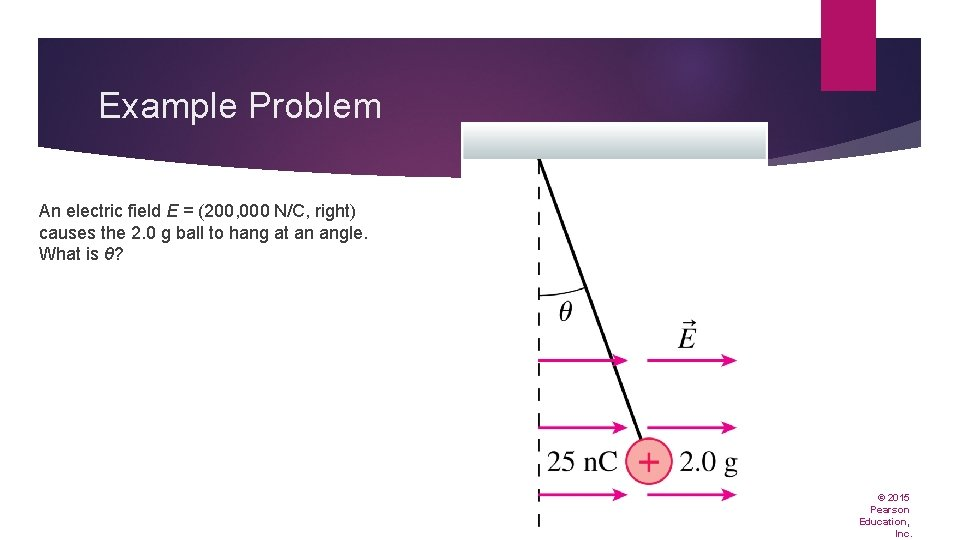Example Problem An electric field E = (200, 000 N/C, right) causes the 2. 0 g ball to hang at an angle. What is θ? © 2015 Pearson Education, Inc.Electric Field Lines Text: p. 651 © 2015 Pearson Education, Inc.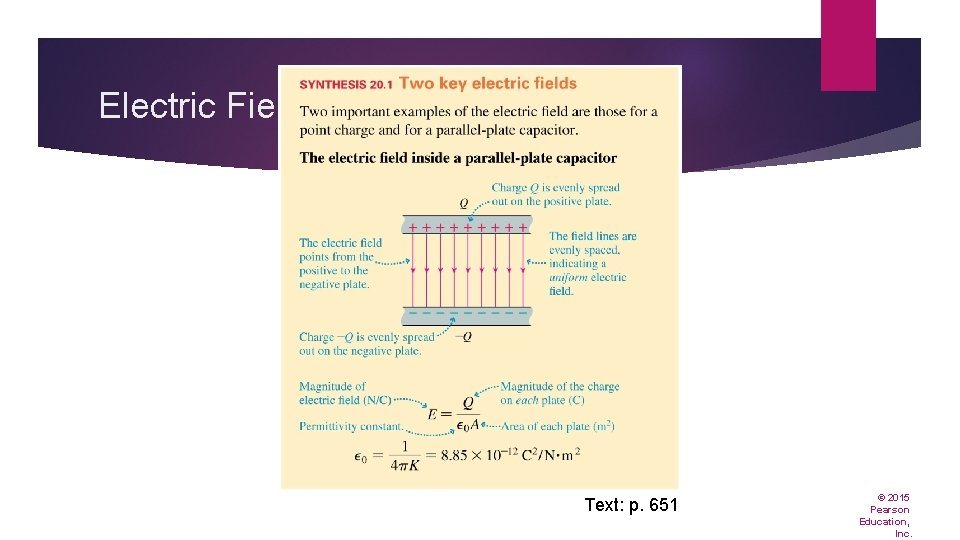Electric Field Lines Text: p. 651 © 2015 Pearson Education, Inc.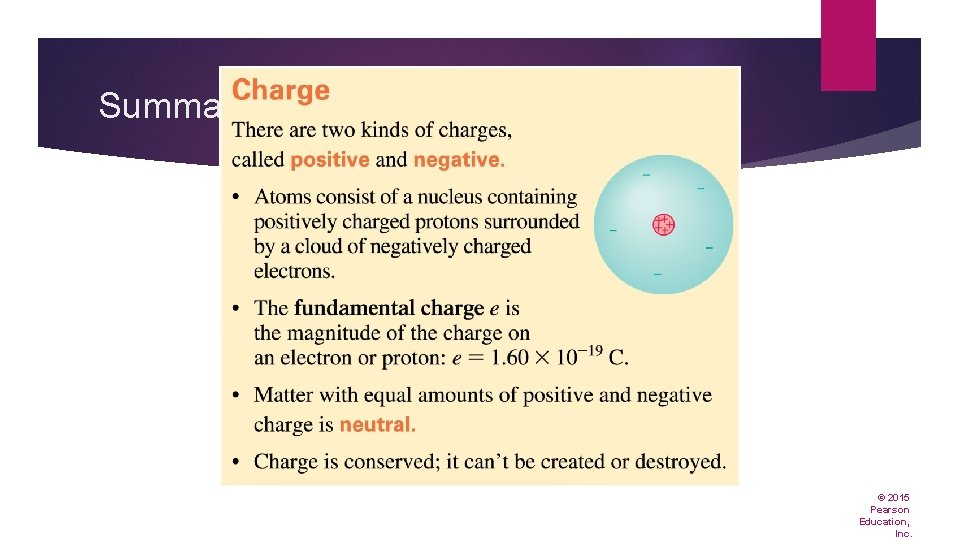Summary: General Principles © 2015 Pearson Education, Inc.Summary: General Principles © 2015 Pearson Education, Inc.Summary: Important Concepts © 2015 Pearson Education, Inc.Summary: Important Concepts © 2015 Pearson Education, Inc.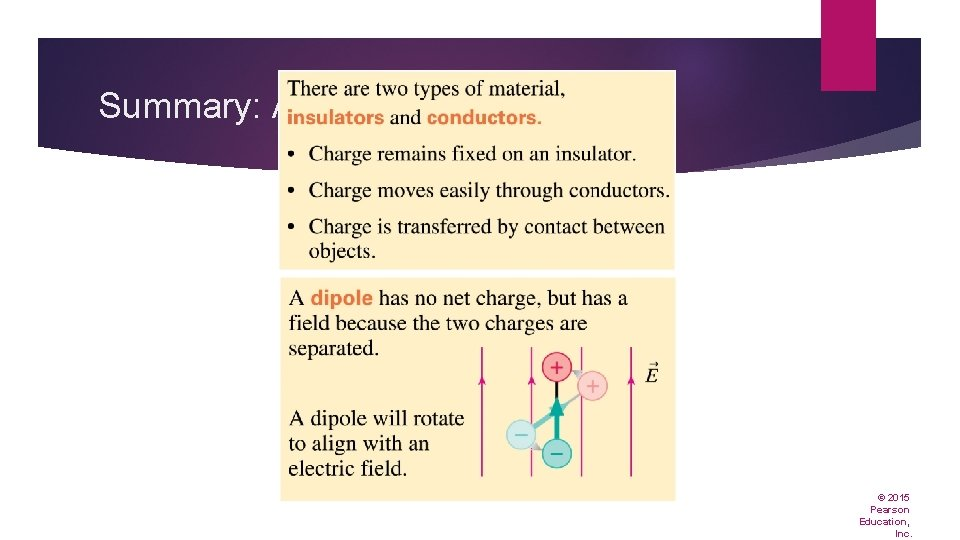Summary: Applications © 2015 Pearson Education, Inc.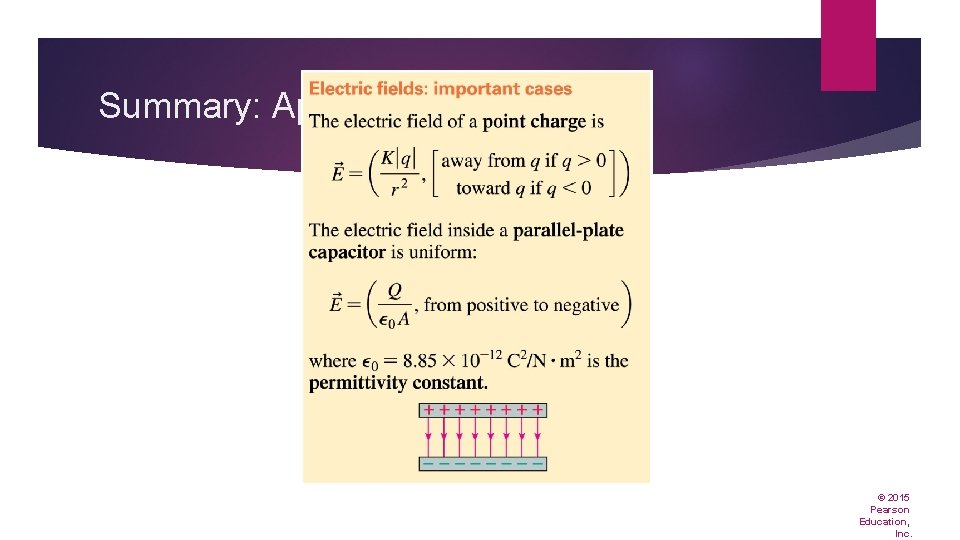Summary: Applications © 2015 Pearson Education, Inc.Summary: Applications © 2015 Pearson Education, Inc.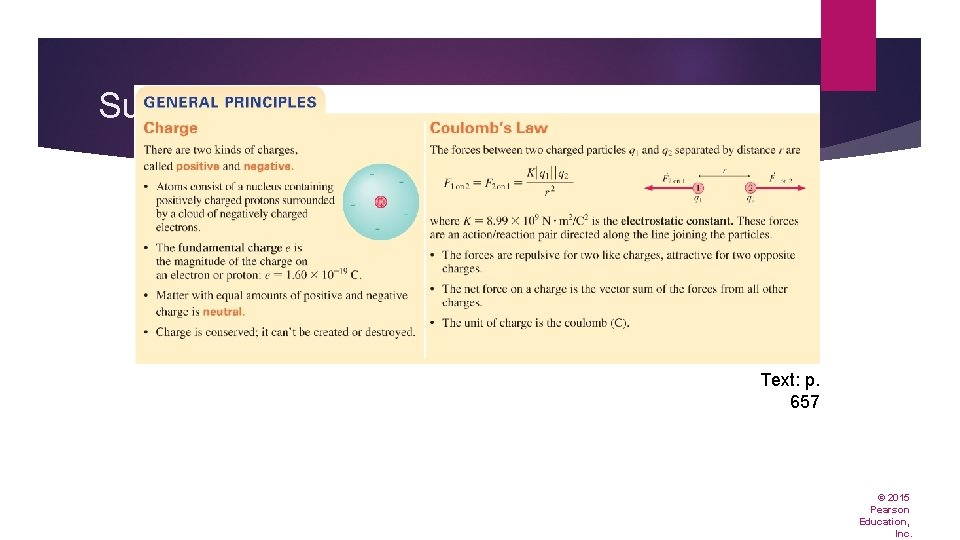Summary Text: p. 657 © 2015 Pearson Education, Inc.Summary © 2015 Pearson Education, Inc.